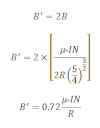# Helmholtz’s Coil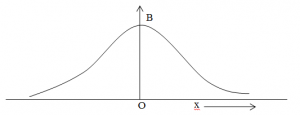Consider a circular current carrying coil carrying current I. The magnetic field at any axis at a distance x from the centre of the coil is: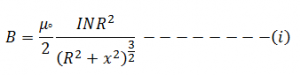From equation-(i) it is seen that the magnetic field varies whith varying a distance from centre to at any point along the axis. It is seen that from above graph the magnetic field due to single circular current carrying coil cannot be uniform.

Helmholtz found that when two identical co-axial circular current carrying coil carrying current in same direction are placed at a distance which is equal to the radius of the coil, the magnetic field due to coil is uniform. It is due to magnetic field of one coil balanced by the magnetic field of other coil. i.e. if the magnetic field of one coil is decreased then the magnetic field of other coil is increased and the net magnetic field remains constant.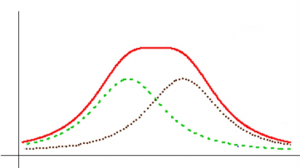Magnitude of B:
The magnetic field due to circular current carrying coil having n number of circular turns carrying current I at a distance x from the centre of the coil is:The rate of increasing or decreasing of magnetic field is given by differentiating b with respect to x, we get,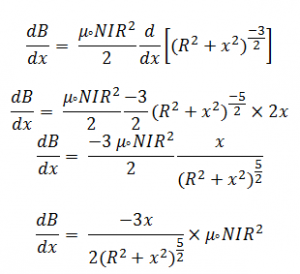Since the magnetic field is uniform then dB/dx is constant.

Now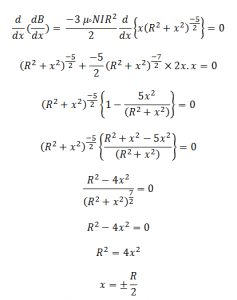The magnitude of magnetic field is maximum in midway of the space between two current carrying coil.

We know that,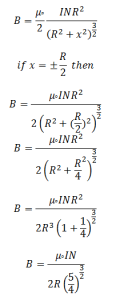Total magnetic field is: